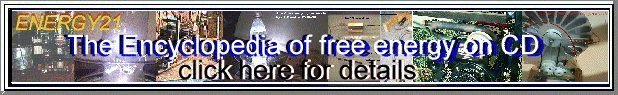Content on this page supplied from the web site of Jim Lux

## ElectroOptical Theory

### Kerr

The Kerr effect results from the impressed electric field causes the assymetric molecules of the liquid to align with the field. This causes the liquid to become anisotropic and birefringent. The change in index is given by:

no-ne = K * E2 * lambda

where:
lambda is the wavelength of the light
E is the electric field strength
K is the Kerr constant

A Kerr Cell is a cell containing the liquid (typically Nitrobenzene) between two flat parallel plates spaced several millimeters. A fairly high voltage (typically 10-20 kV) is placed on the plates..

If the field is such that the cell retards the extraordinary ray by a half wavelength, the polarization rotation will be 90 degrees. If a pair of polarizers is put around the cell, oriented at 45degrees, the assembly acts as a shutter. The voltage required to do this is called the "halfwavelength voltage".

At the half wave voltage, the following is true.

(no-ne)*d = lambda / 2

where
d is length of cell
other variables as above.

Note that the wavelength cancels out when rearranging and substituting to give:

Ehalfwave = sqrt( 1/ (2 * d * K))
for calculating the halfwave E field

d = 1 / (2 * K * E^2)
for calculating required length of cell

#### Sample Kerr Constants

 Nitrobenzene 2.4E-10 cm/V2 Glasses 3E-14 to 2E-23 cm/V2 Water 4.4E-12 cm/V2

For Nitrobenzene (K=2.4E-10 cm/V2 ) and 30 kV/cm (breakdown of air), d = 2.3 cm

### Pockels

(no-ne) = pE

where E is the applied field, and p is a proportionality constant:

A similar calculation to that for Kerr cells can be made to determine the half wave voltage for the cell.

 KDP (Potassium Dihydrogen Phosphate) 3.6E-11 meter/Volt Deuterated KDP (KD*P) 8.0E-11 meter/Volt Lithium Niobate (LiNbO3) 3.7E-10 meter/Volt

Rotation = VBl

where
V is Verdet Constant
B is the magnetic field strength
l is the length

#### Verdet Constant (if l in millimeters, B in Tesla)

 fused quartz 0.004 dense flint glass 0.11 Benzene 0.0087

A peculiarity of Faraday rotation is that it rotates the same direction (e.g. Clockwise or Counterclockwise) no matter which direction the light is travelling. This can be used to make a one way light valve with two polarizers set at 45 degrees to each other.

Copyright 1998, Jim Lux / eotheory.htm / 8 March 1998 / Back to HV Home / Back to home page / Mail to JimWeb www.IceStuff.com